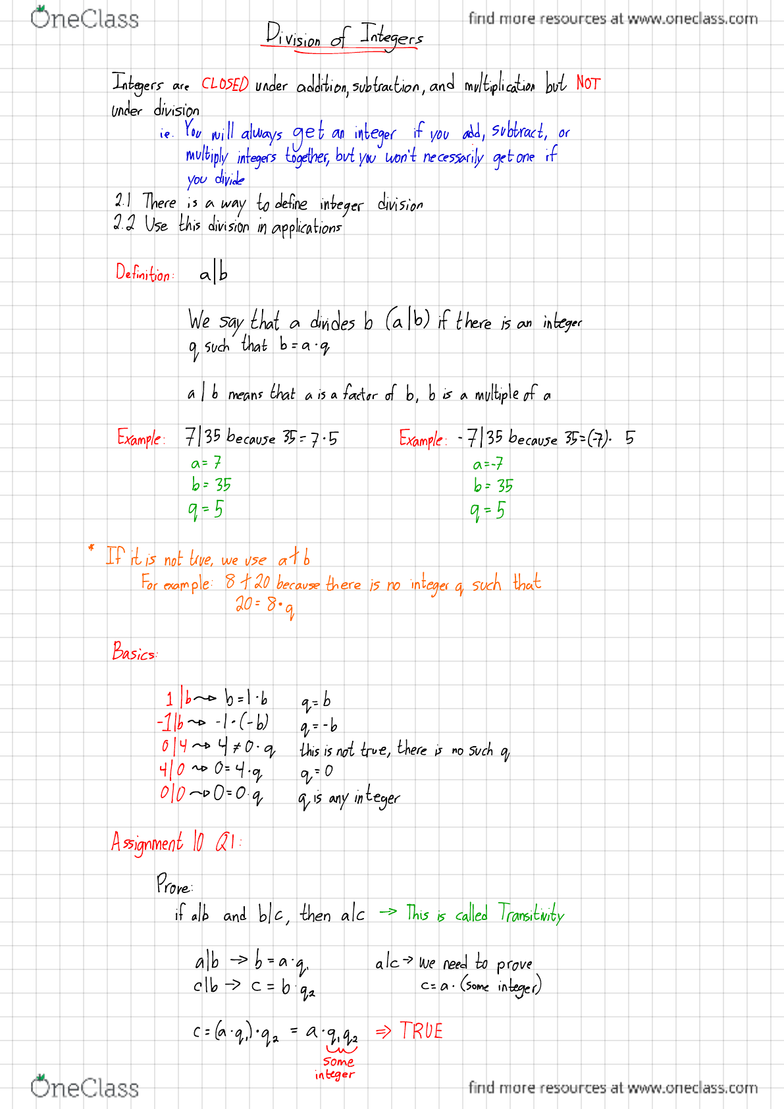Class Notes (1,100,000)
CA (620,000)
McMaster (50,000)
MATH (3,000)
Lecture 17

# MATH 1C03 Lecture 17: Lecture 17

Department
Mathematics
Course Code
MATH 1C03
Professor
Miroslav Lovric
Lecture
17

Page:
of 2Diviionotyintgs
Integers are CLOSED under
addition
,subtraction ,and multiplication but NOT
under division
ie .
You will always get an integer if you
aid ,subtract ,or
multiply integers together,but you won't necessarily get one if
you divide
2.1 There is away to define integer division
2.2 Use this division in applications
Definition :a|b
We say that adivides b(alb)if there is an integer
of such that b=a .q
al bmeans that ais afactor of b,
bis amultiple of a
Example :7135 because 35=7.5 Example :
-7135 because
35=(7
).5
a=7a=
-7
b=35 b=35
q=5 q=5
*If it is not true,we use at b
For example :8+20 because there is no integer qsuch that
20=8 .
q
Basics :|hob =L 'bq= b
11 b- o.1.C- b) q= -b
014 4FO .qthis is not true ,there is no such q
+10 no 0=4 .qq= 0
010-00=0 .qqis any integer
Assignment 10 Ql :
Prove:
if alb and blc ,then ak This is called Transitivity
alb b--a.q.akwe need to prove
clb C=b.qz c= a.(some integer)
C=(a-
qloq ,
=a.qq ,󲍻TRUE
x
ome
integer
If alb and ak then albxtcy for any integers xand y
b= a.q ,bxtcy =a.some integer
c= a.qs (aq ,)x +(aq ,)y=a .some integer
a(qx+ qzy )=a.some integer
qx +gay is some integer so it's TRUE
When we have aremainder :
'Yy =,}#quotient
±a-
remainder
13/4=3 +'F 󲍻13=3.4 + 1
Lquotient [remainder
Division Algorithm :For %,assume b>0 .If a ,b are integers and
b>0 .there exists aunique qand rsuch that
a=bq+r and Oereb
quotient demander
thtswgeunaersanlees
Example:
0loot =105 .lbqtr OE re 16
#
05 105=16161+9 q=6 .
r.-9
96
q
!2!-TT -105=16 .q+r ire 16
-105=16 ff )-9+16-16 c- add tb -b
=Ibl .At 7because rmust be
q= -7OERE 16
r=7
3013¥
to -lyfn -113=37 .qtr Oer ?37
113=37 .qtr are 37 -113=371-3 )-2+37-37
113=37.3+2 q=3 r=2 -113=37 .(4) t35
q= -4 r=3
?